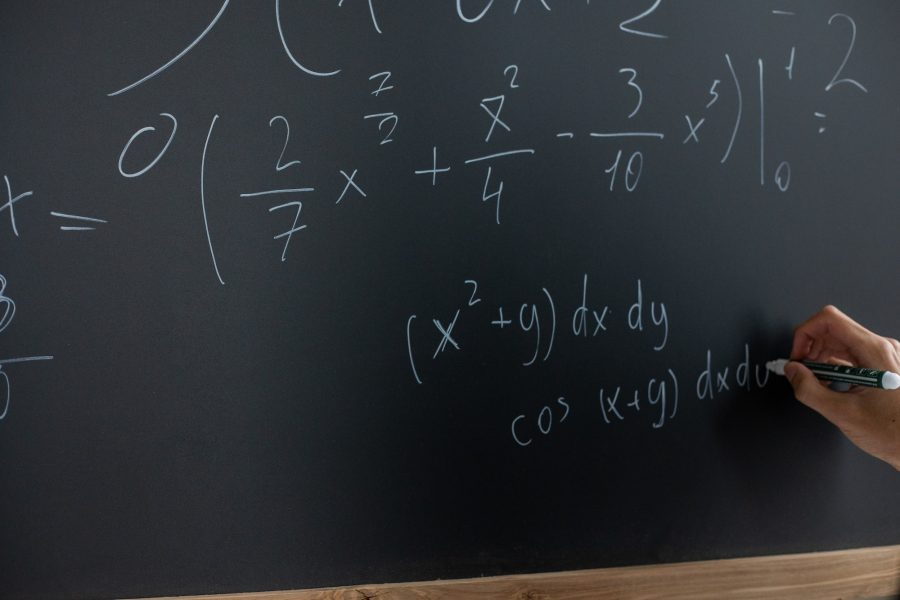# Importance of Algebra in Secondary Math (Sec 1 to Sec 2)

## 15 Dec Importance of Algebra in Secondary Math (Sec 1 to Sec 2)The concept of algebra has several applications in life, especially in the study of sciences and mathematics. It is also considered one of the most important concept in Mathematics.

In lower secondary mathematics, algebra is categorised into several main topics which serve as
important prerequisite for the Math and Science subjects in the N level and O level. These topics
are:

1. simultaneous equations
2. factorisation
3. expansion
4. algebraic fractions
7. direct and inverse proportions

The above topics aim to build a strong foundation in solving algebraic equations, simplifying
algebraic expressions and solving problem sums with unknown variables. It also helps to develop
skills such as critical thinking, logical reasoning and calculation accuracy. For example, students are
tasked to find an unknown parameter such as a speed of a moving car or to form an equation to
describe a number pattern. Therefore, a strong understanding of algebra will equip the students
with valuable skills and renewed confidence in their course of study.

The knowledge learnt in lower secondary Math will be applied in upper secondary Math. Several
chapters in Elementary Mathematics, Additional Mathematics, Physics in O level and N level require
the use of algebra to solve various questions. For example, differentiation and integration (calculus)
which comprises of nearly half the syllabus in Additional Mathematics rely heavily on the knowledge
of solving and simplification of algebraic equations and expressions. As such, having a strong
foundation in algebra at the lower secondary level will assist the students greatly in grasping
concepts at the upper secondary level.

Algebra can also be applied in real life. Algebra promotes logical thinking when it comes to problem
solving. This skill can be applied in the workplace as well. A civil engineer might use the concept of
algebra to determine the correct dimensions of structures to be aligned, a logistics manager may use
algebra to sort and express items and goods in an excel sheet and a finance manager or banker may
use algebra for statistical analysis. The list goes on and on but one thing that is for sure – algebra is
an important mathematical concept that will be applied in many industries in the working world.

In conclusion, algebra in lower secondary mathematics serves as a benchmark for building a strong
foundation in mathematics. This in turn will benefit the student in understanding the upper
secondary Math and Science subjects. Algebra promotes critical and logical thinking when it comes
to problem solving. Not only can algebra be used in school, it can also be applied in the workplace in
several industries such as engineering, life sciences, healthcare and finance. Therefore, algebra is an
important concept and it is highly advantageous to get a strong grasp in algebra in the foundation
years of Sec 1 and Sec 2.

Einstein Takeaway
Never neglect Math in Sec 1 and Sec 2 as all the topics are inter-linked and these topics will continue to resurface in Sec 3, Sec 4 and even the tertiary studies. There is a systematic and effective study strategy to master algebra in lower secondary Math. Let Einstein join you to learn the rope in algebra and develop progressively your interest and proficiency in Math. Contact us now!